# Catch up on the latest AI articles# Deep Learning Is Changing The Way We Predict The Future

3 main points
✔️ An overview of how deep learning is being applied to predict time-series data
✔️ Explain hybrid models as an emerging trend
✔️ Further approaches related to interpretability, factual and non-factual prediction will be discussed

written by Bryan LimStefan Zohren
(Submitted on 28 Apr 2020 (v1), last revised 27 Sep 2020 (this version, v2))
Comments: Accepted by Philosophical Transactions of the Royal Society A 2020

Subjects: Machine Learning (stat.ML); Machine Learning (cs.LG)code：## first of all

Prediction is the twin of anomaly detection in time-series data processing. In this survey paper, we review representative papers that use deep learning to predict time series data. It is widely accepted that traditional methods are more effective, and we clarify why this is the case and explain the emerging trends.

Time series modeling has historically been a major area of academic research. It has formed an indispensable application in topics such as weather, bioscience, pharmaceuticals, and decision-making in retail and financing. In contrast to traditional methods (autoregressive, exponential smoothing, structural time series models) that create parametric models based on the findings of domain experts. modern machine learning models take a purely data-driven approach to learning temporal dynamics. As data becomes more abundantly available and computational power increases, machine learning has become an integral part of the next generation of time series forecasting models.

## SOTA Technology

The diversity of time series problems across different domains has led to the emergence of numerous neural network design alternatives. In this paper, we summarize common methods for time series forecasting using deep learning. Since most of the methods discussed are well known, we will focus on what is written about their features, limitations, and contrasts with other methods when applied to time series forecasting, rather than the mathematical formulas.

First, we will discuss one-step-ahead, which is a univariate method for forecasting the next point in time based on past data, but it can be easily extended to multivariate. The extension to multi-horizon forecasting will be described later.

An overview of deep learning includes automatic model parameter selection, traditional machine learning such as kernel regression, support vector regression, In addition, Gaussian processes are often used for time series prediction. It has been extended to deep Gaussian processes. Older models of neural networks have also historically been used for time series applications.

### basic building block

In its simplest case, the one-step-ahead prediction model takes the following form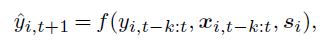where $\hat{y}_{i, t+1}$ is the model's prediction, $y_{i,t-k;t}={ y_{i,t-k},\cdots , y_{i,t}}$,$x_{i,t-k;t}={ x_{i,t-k},\cdots , x_{i,t}}$ is the target and extrinsic input look observations over the lookback window (lookback window) k, and $s_i$ is the static metadata associated with the entity, such as the sensor location. And f() is the prediction function learned by the model. Deep learning, when applied to time series, can be seen as encoding the relevant past information into the latent variable $Z_t$.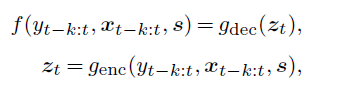$g_{enc}, g_{dec}$ are encoder and decoder functions, respectively, and constitute the basic building blocks of deep learning architecture. The encoder can have the structure of (a), (b), and (c) in Figure 1.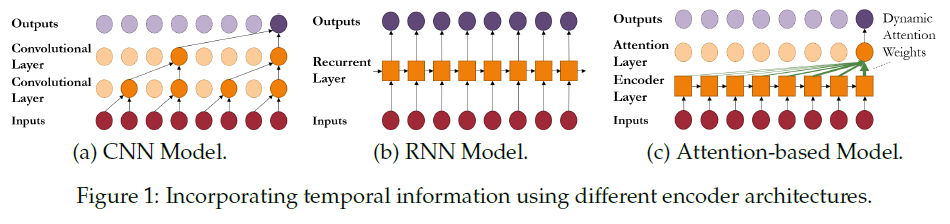Convolutional Neural Network (CNN) CNN (Convolutional Neural Network)

When applying CNNs to time series data, we take the structure of a multi-layer causal convolution [32, 33, 34]. This is because only past information is used. The intermediate feature values of the hidden layer are as follows.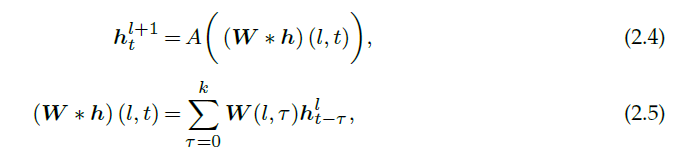Looking at the one-dimensional case, it is analogous to Finite Impulse Response (FIR). This has two implications. First, the relation is invariant. Second, the time window width is constant. The size of this lookback window or receptive field must be carefully set so that all relevant historical data can be captured. A single-layer causal CNN combined with a linear activity function is equivalent to an AR (Auto-regressive) model.

Dilated Convolution Layers [32, 33] are used to reduce the computational burden of incorporating long-term data. to reduce the computational burden of incorporating long-term data.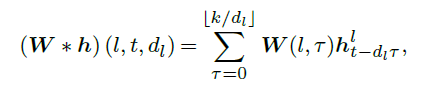[] is the truncation function and $d_l$ is the dilation rate per layer. It can be seen as a convolution with a lower sampling rate for lower layers. It is more efficient than normal causal convolution to use past data.

RNN (Recurrent Neural Networks)

RNNs have been used to model sequences for a long time. Many models have been developed for time series prediction as well. [37, 38, 39, 40] As a core, it has an internal memory state.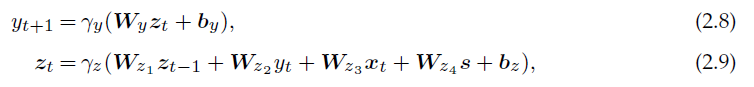Note that in RNNs, you do not have to explicitly determine the length of the lookback window.

In comparison with signal processing, it is similar to the nonlinear IIR (Infinite Impulse Response). In RNN, there is a problem of gradient explosion or disappearance, which can be seen as a resonance of memory state. LSTM uses a "cell state" to store long-term information to deal with this problem.

In this paper, LSTM only lightly explains the structure, but I would like to introduce another paper that strongly discusses the superiority of LSTM." In another paper, " A Comparative Analysis of Forecasting Financial Time Series Using ARIMA, LSTM, and BiLSTM," the authors show that LSTM has better long-term forecasting accuracy and 88% lower errors on average than ARIMA or its analogs. In addition, BiLSTM is a bi-directional learning method. BiLSTM, which learns bi-directionally, reduces the error by 93% compared to ARIMA, and BiLSTM takes more epochs to reduce the loss than LSTM and extracts features that LSTM cannot.

In a Bayesian filter, such as the Kalman filter, the inference is using state transitions and error correction steps, and done by updating a series of latent states with sufficient statistics; RNNs can be seen as approximating both of these steps simultaneously since the memory vector contains all the relevant information needed for prediction. [39 ]

Attention

The attention mechanism uses dynamically generated weights to aggregate temporal feature values. It allows you to focus directly on salient points in the past, no matter how far back in time you go. Multiple attention layers can be used simultaneously; in one example, attention aggregates feature values extracted by an RNN encoder. [52 ] A transformer structure is also considered, which has two advantages: first, any event can be directly mentioned, and second, by using attention weight patterns carved out for each type of phenomenon, temporal dynamics specific to each type can be learned.

Loss function

The neural network can handle both discrete and continuous values. The decoder and the output layer of the network are matched to the desired type of the target value. The estimation methods include point estimation and probability estimation.

##### Point Estimates

Here are the equations for classification and regression.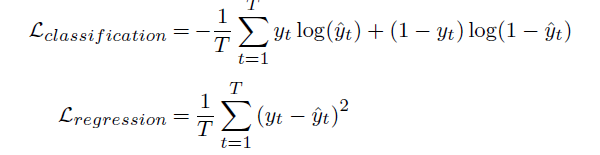##### Probabilistic Outputs

Outputs the probability distribution at each output using the Softplus function or other functions.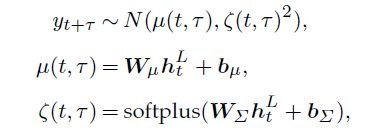Understanding the uncertainty of the model can be useful for decision-making in different domains.

### multiple time prediction method

Iterative Methods Iterative Methods

Typically, an autoregressive deep learning structure is used for multi-point prediction, where the target value can be regressively fed to the input at the next point in time, as shown in Figure 2 (a). This method is simple but has the risk that errors at each time point will accumulate and produce large errors.

Direct Methods

To solve the above problem, the direct method uses all inputs directly. Usually, Seq2Seq sequences are used. There is also a simple method that directly generates a fixed-length vector for the desired number of prediction time points. However, you need to set the maximum prediction range.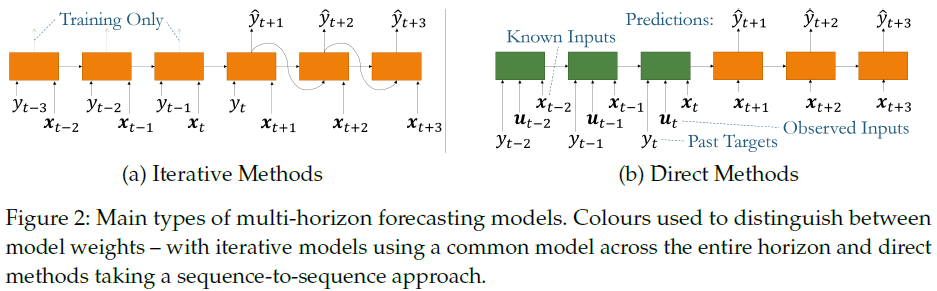## Capturing domain knowledge in a hybrid model

Despite its popularity, the effectiveness of machine learning on time series forecasting has historically been questioned. One example is forecasting competitions such as M-competition. The prevailing wisdom is that state-of-the-art methods are not capable of beating high accuracy forecasts, but rather that ensembles with simple models tend to produce better results.[59, 62, 63].

Two main reasons have been identified that explain the poor performance in machine learning. First, the flexibility in machine learning methods is a double-edged sword, making them prone to overfitting.[59 ] Second, similar to the stationarity requirement in statistical models, machine learning models are sensitive to how the inputs are preprocessed.This ensures that the data distribution is the same during training and testing.

A recent trend is the development of hybrid models. They address the above two limitations and show improved properties in various applications compared to pure statistical and machine learning methods. [38, 64, 65, 66 ] Hybrid methods combine well-analyzed quantitative time series models with deep learning. There, a deep neural network is used that generates model parameters at each time step. The hybrid model, on the other hand, allows domain experts to train the neural network with known information. This reduces the assumed space of the network and facilitates generalization.

### Non-stochastic hybrid model

In parametric time series models, the forecasting equation is usually analytic and outputs a point estimate. In a non-probabilistic hybrid model, such a prediction equation is modified to combine statistical and deep learning elements.

The ES-RNN (Exponential Smoothing RNN) updates the Hult-Winters exponential smoothing model by combining the multiplier level and seasonality factors with the output of deep learning, as shown in the following equation.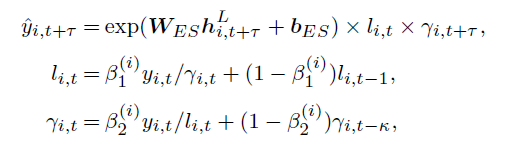### Stochastic Hybrid Model

Stochastic hybrid models are used in applications where distributional modeling is important. Stochastic generative models are used for time kinematics such as Gaussian processes and linear state-space models. Rather than updating the prediction equation, We use a neural network to generate parameters that predict the distribution at each step.

For example, a deep state-space model encodes the time-varying parameters of a linear state-space as in the equation below and infers them through a Kalman filter.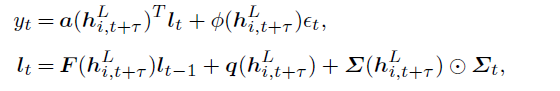## Using deep learning to facilitate decision support

While modelers are primarily concerned with forecast accuracy, end users generally use forecasts to guide their future behavior. Even in the early stages, where time series forecasting is essential, a better understanding of the temporal dynamics and motivations behind model forecasting can help users optimize their decision-making.

### Interpretability of time series data

There is a growing need to understand the "how and why" of model prediction when applying neural networks to essential applications. These days, end users have little prior knowledge of the relationships between the increasing size and complexity of data sets. Since standard neural networks are black-box-like, a new research area has emerged on methods for interpreting deep learning models.

The technique of a posteriori interpretation

This model is an interpretation of the learned model. It helps to identify important feature values and examples without changing the original weights. One approach is to apply simpler surrogate models between the inputs and outputs of the neural network, such as LIME (Local Interpretable Model-Agnostic Explanation) [71 ], also known as XAI, SHAP ( Shapley additive explanations) [72 ], among others.

Subsequently, gradient-based methods were proposed, such as Saliency maps [73, 74 ], Influence Functions [75 ]. They analyze the gradient of the network to find which inputs have the most influence on the loss. A posteriori interpretability methods reveal feature value properties, while sequential dependencies between inputs are usually ignored.

Interpretability incorporated by attention weights

The other approach is to design the architecture directly using explainable elements. Usually, the attention layer is strategically incorporated. Analysis of the attention weights reveals the relative weights of the feature values at each point in time. There are examples where the magnitude of the attention weights in a particular example analyzes which time points were most important to the prediction, [53, 55, 76 ] and where the time variation of the distribution of the attention vectors shows consistent temporal relationships such as seasonality. [54 ]

### Factually incorrect predictions, causal inference over time

In addition, deep learning can support decision-making by making out-of-observation predictions or predicting non-factual cases. Predicting non-factual cases is particularly useful for scenario analysis applications. It can be used to predict both the past and the future.

While there are large-scale deep learning methods for estimating causal effects in static settings, a major challenge is the presence of time-dependent confounding effects. This is because of the cyclical relationship, where actions that can affect the objective variable also condition the observation of the objective variable. A straightforward estimate, without adjusting for time-dependent confounding, will produce bias. 

In recent years, several deep learning methods have emerged that adjust for time-dependent confounding. A statistical approach extends the Inverse-Probability-of-Treatment-Weighting (IPTW) method to estimate the probability of applying treatment with a marginal structural model in epidemiology.  In another approach, we extend the G-computation framework to obtain the distribution of targets and actions. [82 ] Additionally, a loss function was proposed that employs adversarial learning of domains. [83 ]

## Summary

With the current availability of big data and increasing computing power, prediction using deep learning is proving successful in many domains. It is necessary to carefully consider the characteristics of each building block. In addition, we have summarized the extensions used for decision making, explainability, and prediction of cases that have not actually happened.

On the other hand, it has its weaknesses. Deep learning usually requires the time series to be separated into a fixed time frame. For data appearing at random intervals, investigations such as Neural Ordinary Differential Equation have been started. Furthermore, time-series data may have a hierarchical structure. The development of a model that explicitly takes such a structure into account is also a future research topic.

If you have any suggestions for improvement of the content of the article,
please contact the AI-SCHOLAR editorial team through the contact form.